Start typing, then use the up and down arrows to select an option from the list.# Calculate Oxidation Numbers

Jules Bruno
257views
1
Like we said before, oxidation numbers don't always correspond to real charges, and therefore a list of rules will be necessary. Now we're going to say when different elements are in a compound, these specific rules will be used to calculate our oxidation numbers. Now here, let's take a look at these specific oxidation number rules. When it comes to Group One elements, they will be plus one when connected to any other element. So that's something similar to charges of groups, and we're gonna see some similarities between oxidation numbers and charges. But then we're going to see a huge deviation from that group. Two A plus two. When they're connected to another element flooring, it's minus one. When it's connected, toe any other element. Now this is where things start to change. Hydrogen can be either plus one or minus one. It's plus one when it's connected to non metals, for example, if hydrogen is connected to glory or hydrogen is connected to oxygen or it's connected to the nonmetal of nitrogen. In all these instances, since hydrogen hydrogen is connected to a non metal, it's oxidation number. We plus one. Now it's negative one. When it's connected toe a metal or borrowing So, for example, and a H or C H two or B H three. In these cases, hydrogen will be minus one for its oxidation number. Now oxygen is even Mawr varied. We're going to say here that oxygen, when it's not a peroxide or super oxide, it's oxidation numbers minus two. When it's in its peroxide form, it's oxidation. Number will be minus one. Now. What exactly is a peroxide? Well, a peroxide, we're going to say, is when you have to Group one elements connected to two Oxygen's So example h Two Hydrogen, which are in Group one egg to oxygen's. This would be hydrogen peroxide ally 202 lithium peroxide or K 202 Potassium peroxide. Now, if oxygen is a super oxide, it's oxidation. Number is minus a half. What is a super oxide? Ah, super oxide is when you have one Group one a element with two oxygen's. So here we could have potassium super oxide CSE, um, super oxide, sodium super oxides. So just remember oxygen. Very soap. Just look be on the lookout. Do you have a super oxide or peroxide? If not it's oxidation. Number is minus two. And then finally, when we're talking about groups seven and we're talking about chlorine, bromine and iodine, they're going to be minus one except when they're connected to oxygen. In that case, we won't know what their new oxidation number will be and will have to calculate it. So again, we use these specific oxidation number roles when we're talking about different elements connected together. So we're gonna have to utilize them to determine the oxidation number of any element given to us within a compound.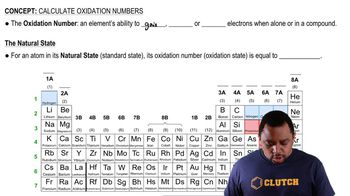02:4201:16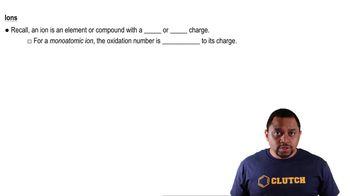01:15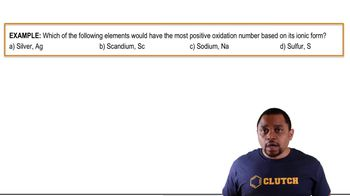01:38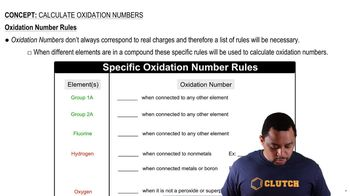03:13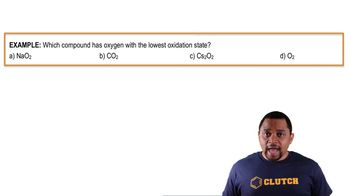01:36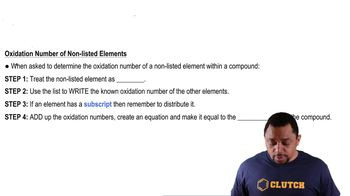01:09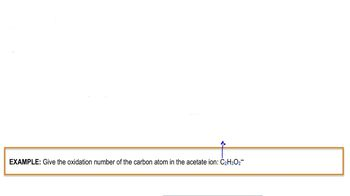01:22